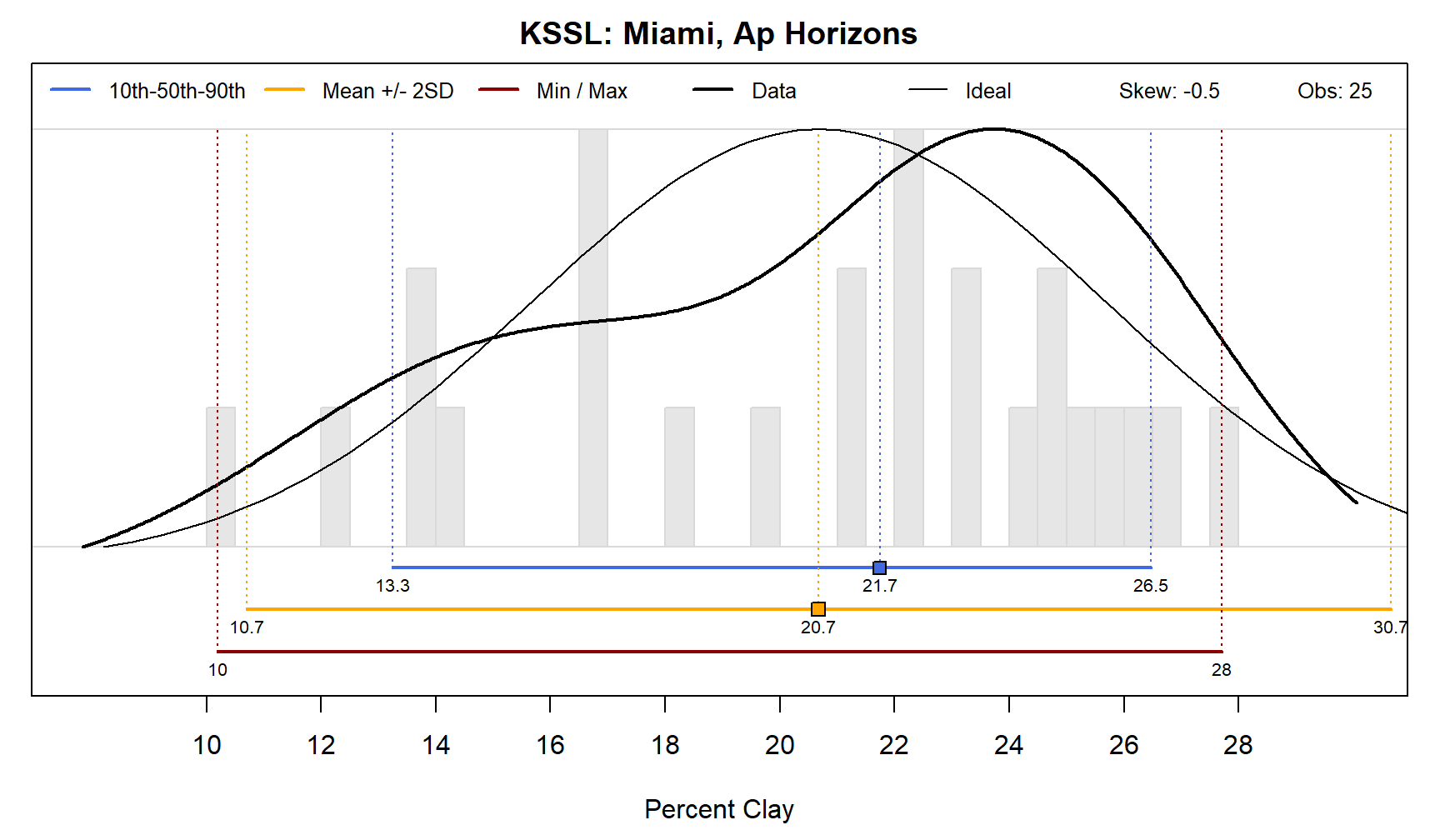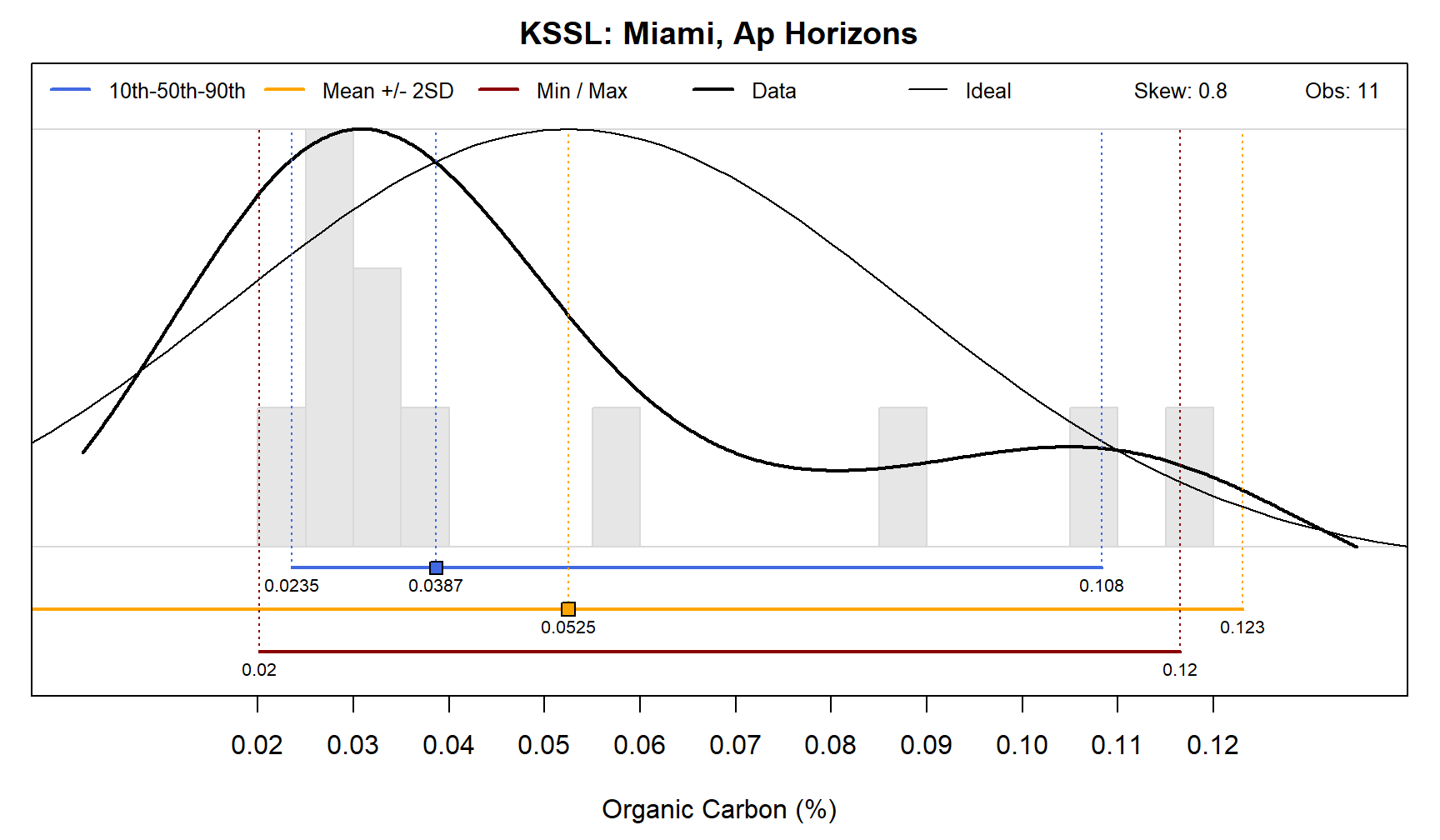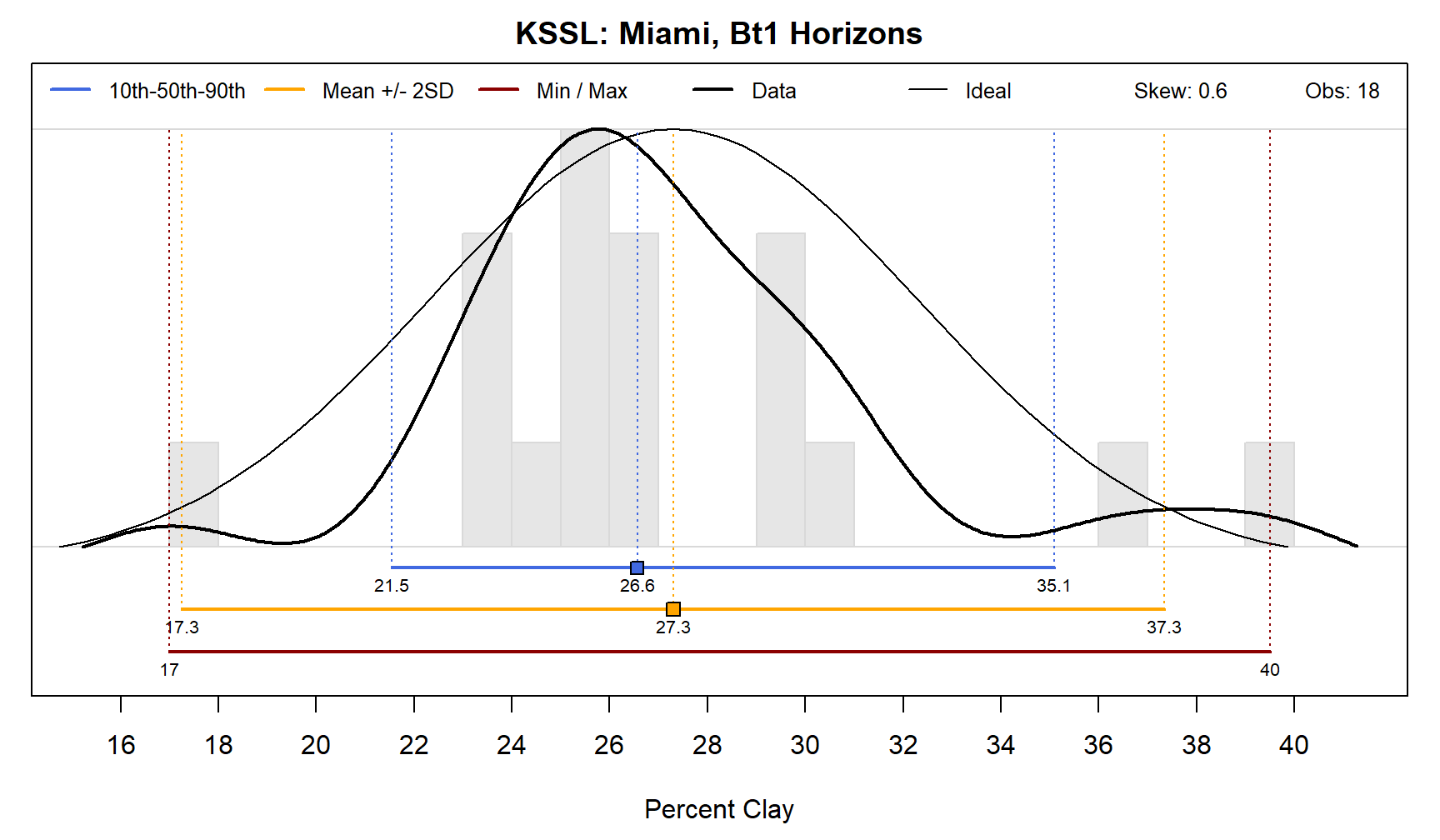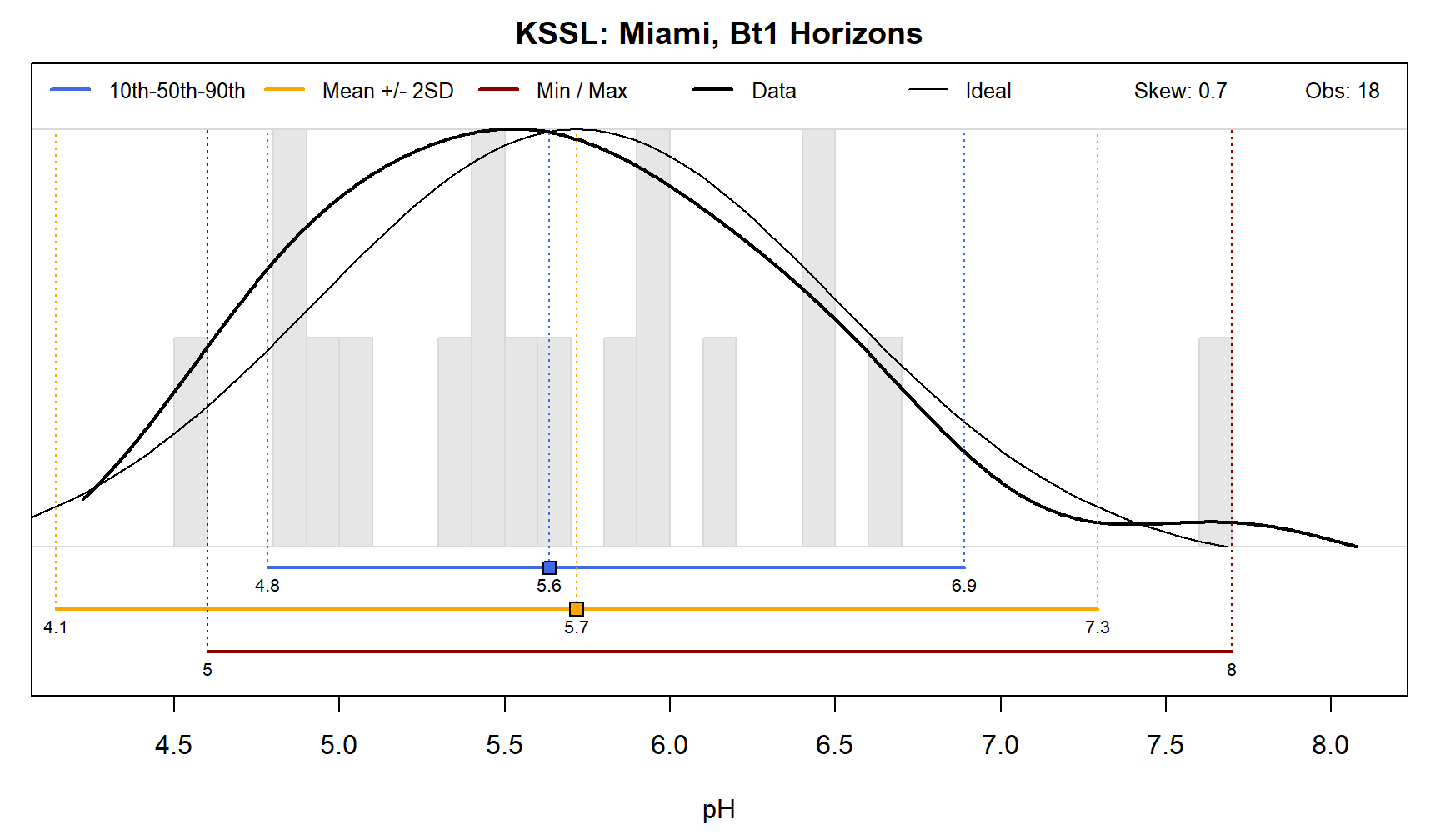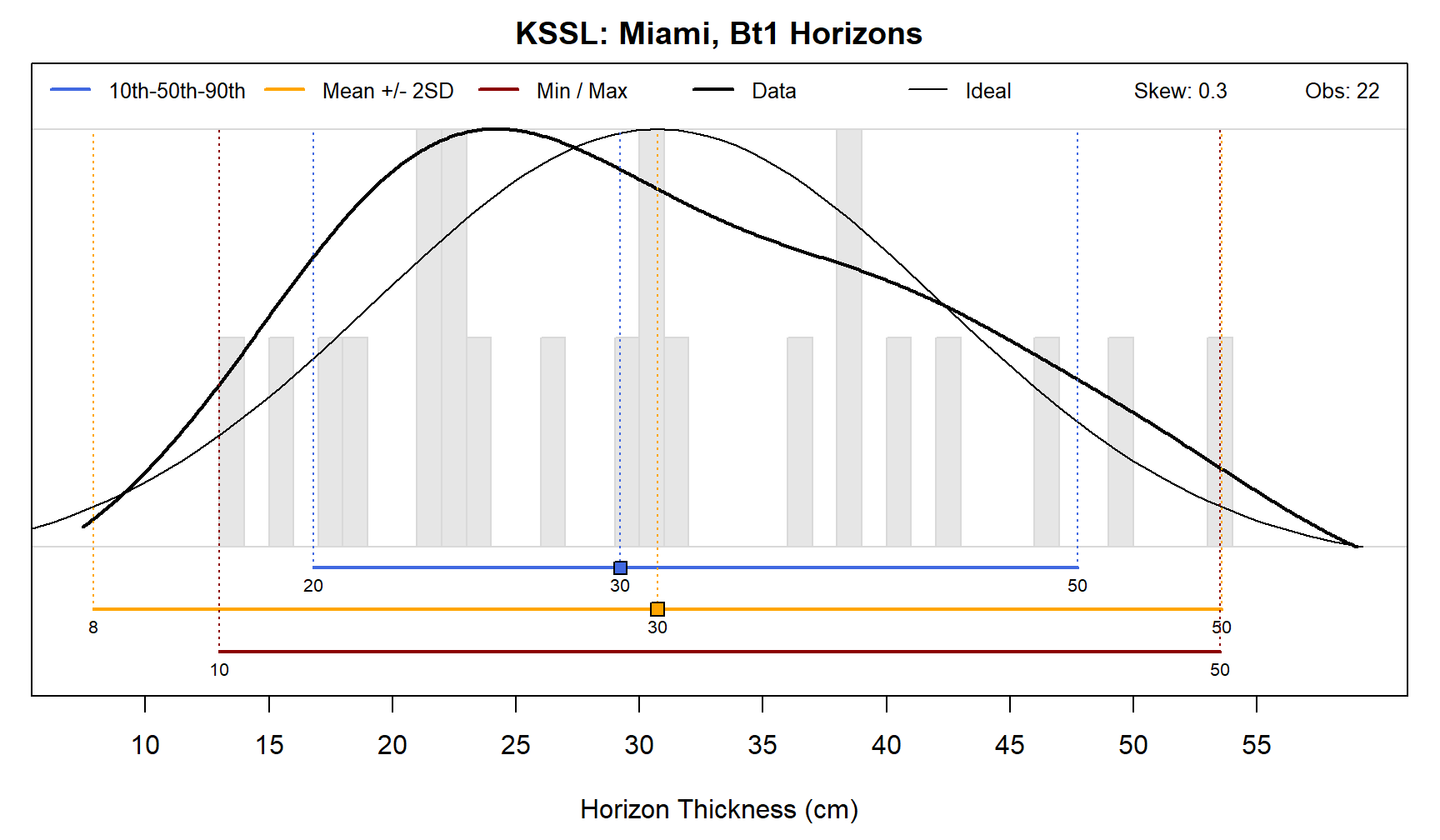# Introduction

This document describes the percentile as a robust measure of central tendency and spread within a distribution of values. Examples are given for its application in the context of soil data summaries.

## Definition and Description of the Percentile

Within a set of data, the n-th percentile describes the value below which n% of the data, when sorted, fall. For example, within the integer sequence spanning from 0 to 100, 50 is the 50th percentile or median, 10 is the 10th percentile, and 90 is the 90th percentile.

Consider the following (hypothetical) field-described clay content from the A horizon of the same taxa:

11, 10, 12, 23, 17, 16, 17, 14, 24, 22, 14

sorted:

10, 11, 12, 14, 14, 16, 17, 17, 22, 23, 24

resulting:

• 10th percentile: 11
• 50th percentile: 16
• 90th percentile: 23

### Visual Demonstration

Consider a histogram derived from carbon stock values representing various regions of the US. Within this distribution a carbon stock of 85 tons/ha is associated with the 16th percentile. In other words, 16% of the collected carbon stock values are less than 85 tons/ha.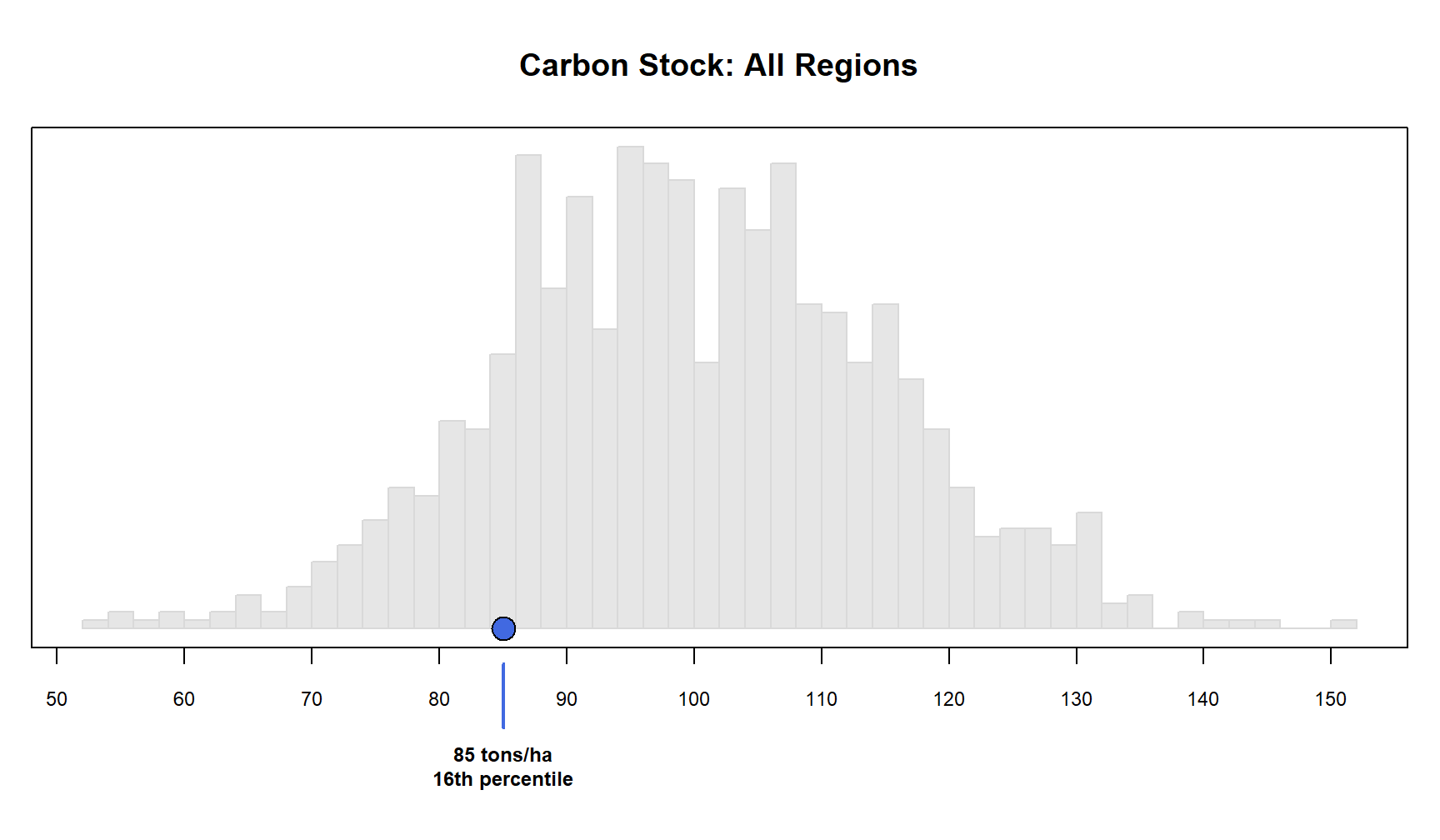## Why Percentiles?

• Percentiles require no distributional assumptions and are bound to the data from which they are computed. This means that percentiles can provide meaningful benchmarks for both normal and non-normal distributions, and, the limits will always fall within the min/max of the observed data.

• Direct interpretation; consider the 10th (P10) and 90th (P90) percentiles: “given the available data, we know that soil property p < P10 10% of the time, and, p < P90 90% of the time”. This same statement can be framed using probabilities or proportions: “given the available data, soil property p is within the range of {P10 − P90} 80% of the time”.

• Percentiles are simple to calculate, requiring at least 3, better 10, and ideally > 20 observations.

• The median is a robust estimator of central tendency.

• The lower and upper percentiles (e.g. 10th and 90th) a robust estimator of spread.

• Statistics such as the mean, standard deviation, and confidence intervals are based on the normal distribution.

## Small Sample Sizes and Interpolation

Estimation of percentiles is based on ranking of the original data. Interpolation between observed values is required when sample size is small (generally less than 10 observations). Consider the values `(1,3,5,6,7,9,9,10)`. Estimation of the 10th, 50th, and 90th percentiles results in 2, 7, 10 respectively. Since we are not typically interested in the estimated percentiles verbatim, the interpolated estimates are close enough. The Harrel-Davis estimator is a robust method for deriving percentiles in the presence of ties and when sample size is small.

# Common Distribution Shapes

The following figures demonstrate the relationship between distribution shape, measures of central tendency (mean and 50th percentile), and measures of spread (mean +/- 2 standard deviations, and 10th / 90th percentiles). Within each figure is an idealized normal distribution that is based on the sample mean and standard deviation. The y-axis can be interpreted as the “relative proportion” of samples associated with a value on the x-axis. The thick, smooth lines represent an estimate of density using real measurements, a continuous alternative to the histogram (grey columns).

## Symmetry Around the Central Tendency

With a large enough sample size, the distribution of some soil properties can be approximated with the normal or Gaussian distribution. In this case, the mean and median are practically equal and the spread around the central tendency is symmetric. Examples include lab measured clay content or pH, from a collection of related samples (e.g. A horizons from a single soil series concept).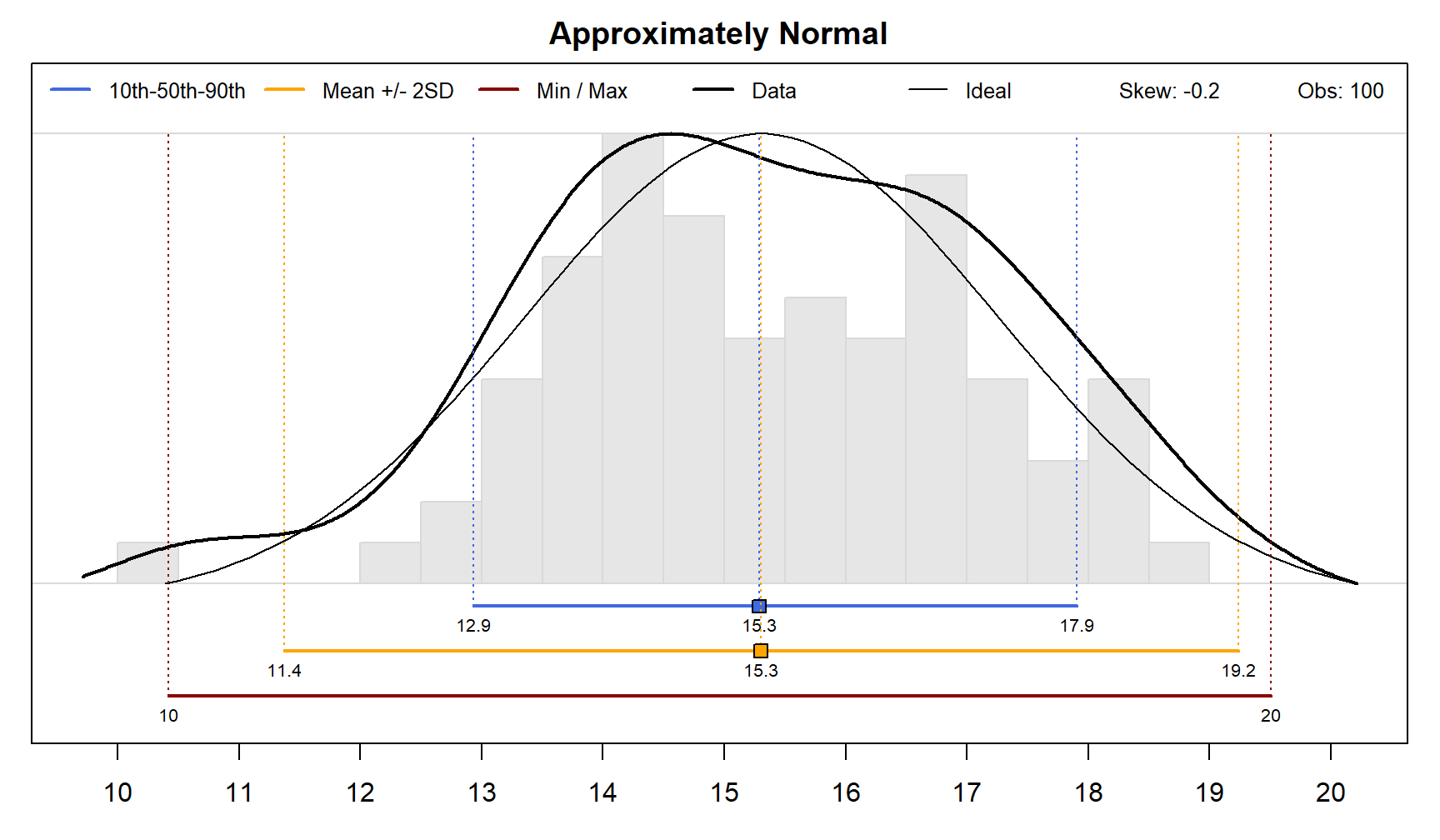## Skewness: Asymmetric “Tails”

Various forms of the log-normal distribution are typically more accurate approximations of soil properties. Log-normal distributions with a “short tail”, or a low degree of asymmetry around the central tendency (skewness), are common. Note the shift between mean and median, and the unequal distances to 10th and 90th percentiles. Examples include lab measured organic carbon and field measured rock fragment volume.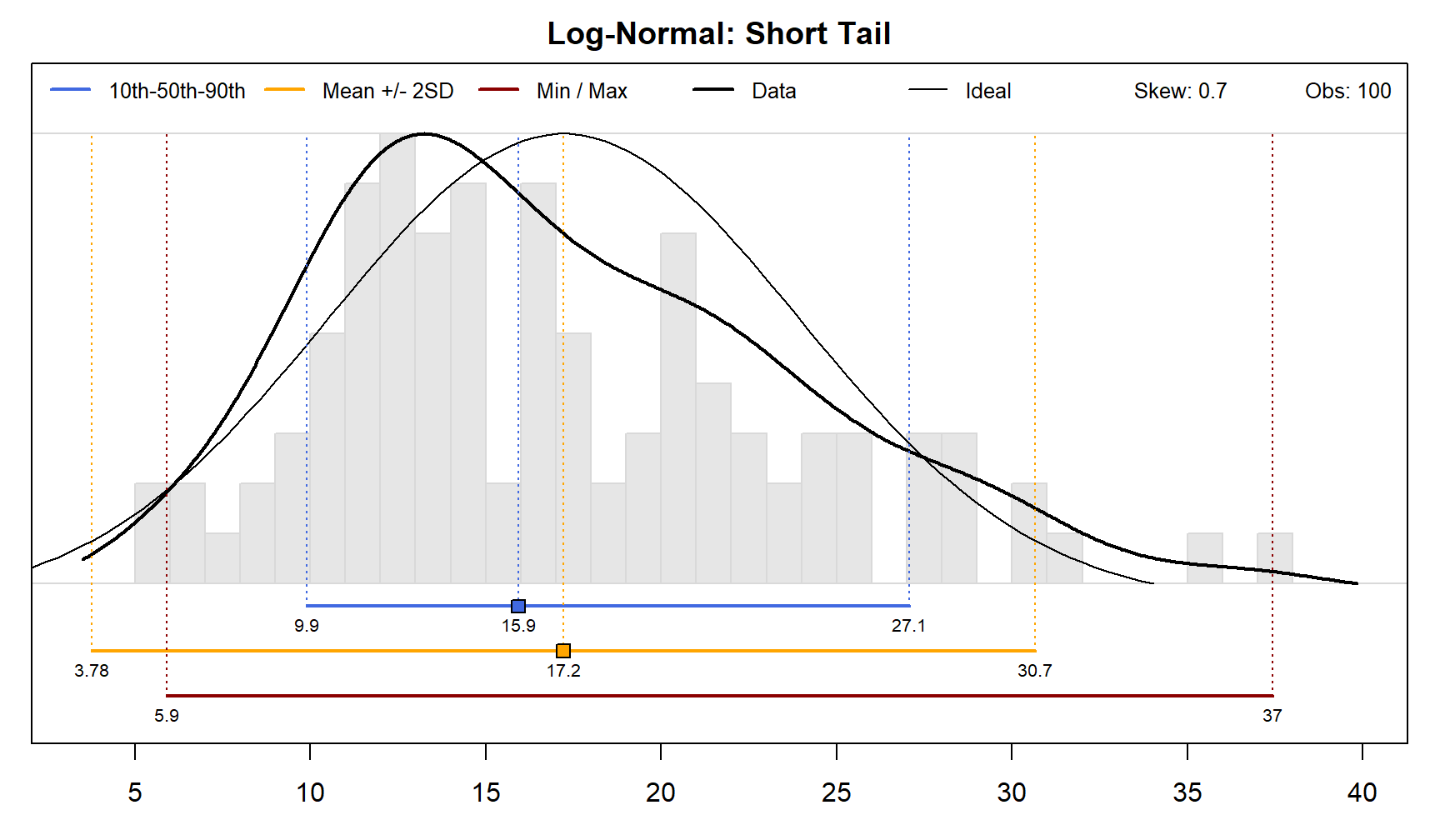min -2SD P05 P10 P50 mean P90 P95 +2SD max sd skew n
5.9 3.8 7.9 9.9 15.9 17.2 27.1 30 30.7 37.4 6.7 0.7 100

Log-normal distributions with a “long tail”, e.g. more skewed, are commonly encountered when summarizing GIS data sources such as elevation, slope, and curvature. Note that the mean +/- 2SD is no longer a meaningful representation of spread around the central tendency.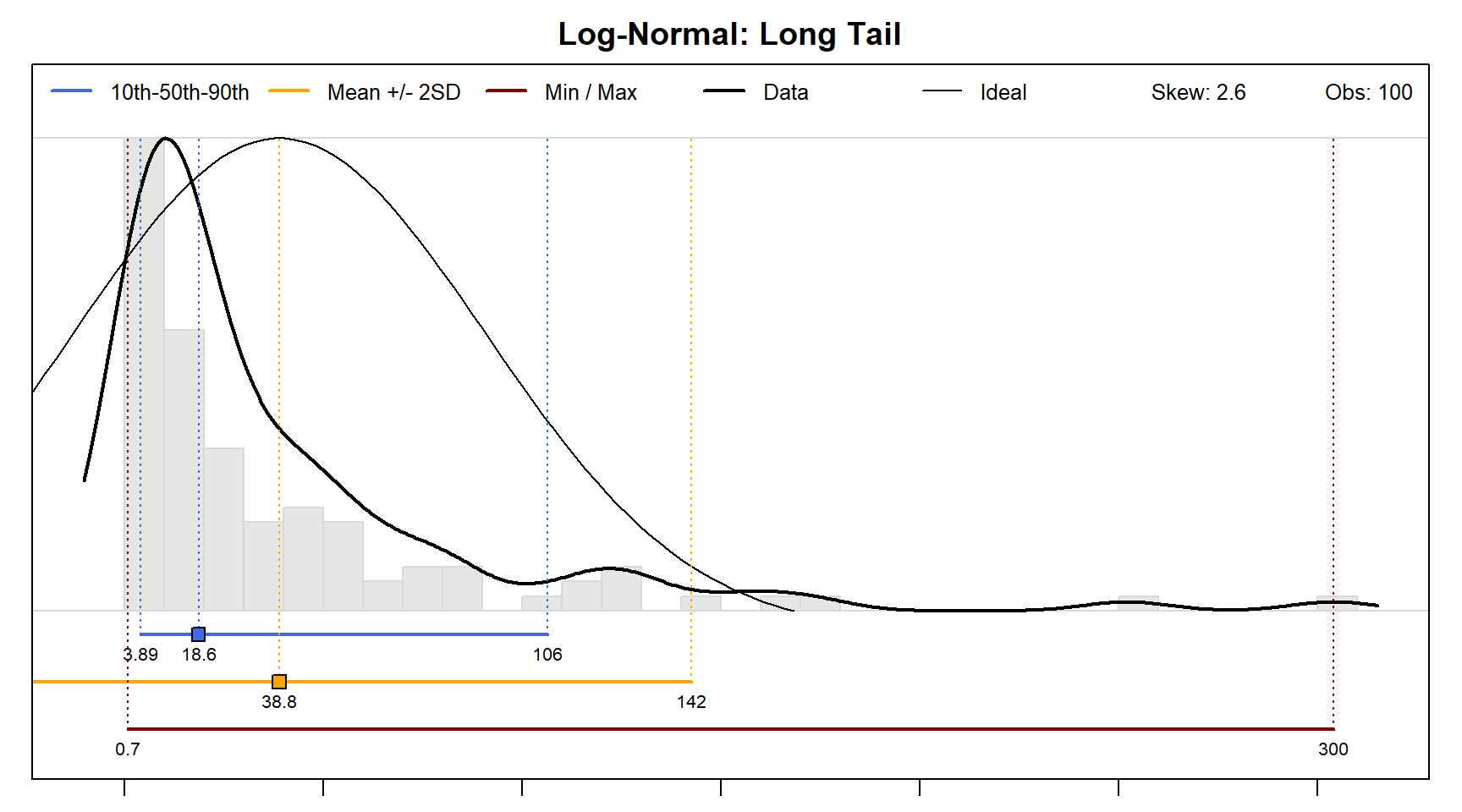In general, the further the departure from a normal distribution, the less meaningful mean and standard deviation are as metrics of central tendency and spread.

## Caution: Comparison of Apples and Oranges

A mixture of incompatible data (e.g. A and Bt clay content) will always result in unreliable summary statistics. Consider the following hypothetical, multimodal distribution of clay content resulting from erroneously combining data from A and Bt horizons. In this case the estimates of central tendency are representative of neither group and the estimates of spread are misleading. A graphical inspection of distribution shape is critical to meaningful estimation of central tendency and spread.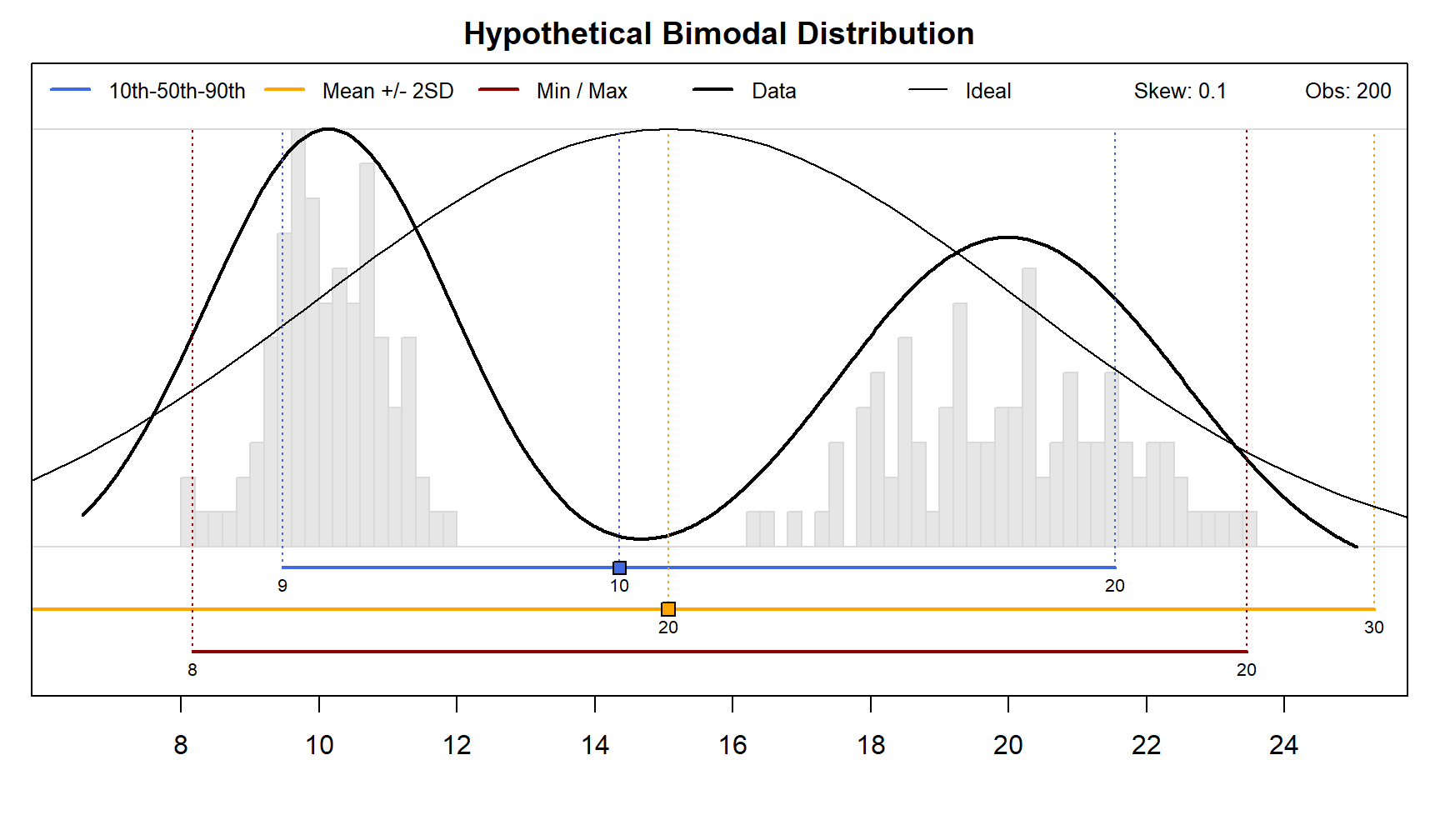# Examples

## Lab Characterization Data

The following examples are based on KSSL data correlated to the Miami series.